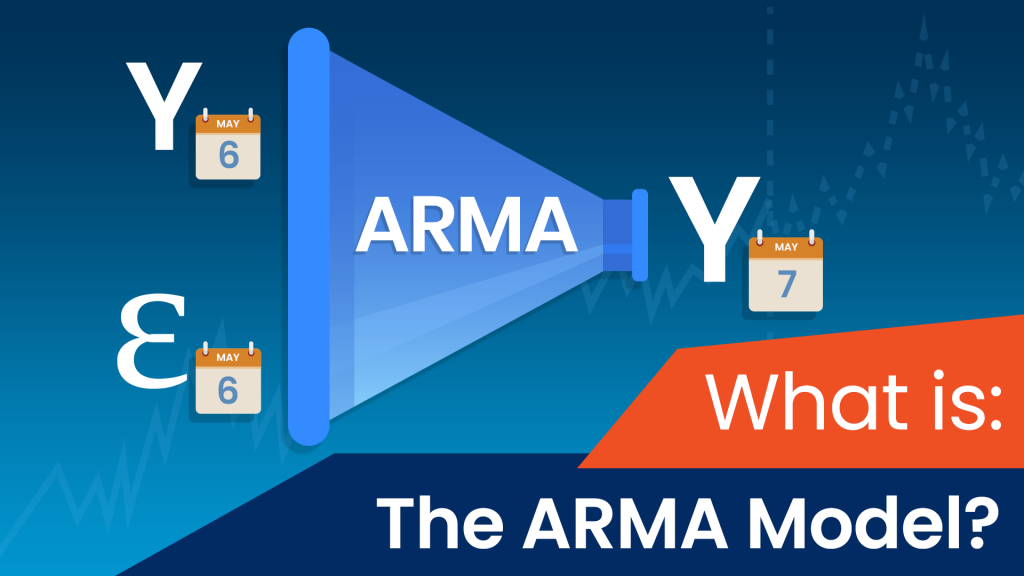Updated on 16 Jul 2021

# What Is an ARMA Model?Viktor Mehandzhiyski
Published on 12 May 2020 4 min readIn time series, we often rely on past data to make estimates about current and future values. However, sometimes that’s not enough. When unexpected events like natural disasters, financial crises, or even wars happen, there can be a sudden shift in values. That's why we need models that simultaneously use past data as a foundation for estimates, but can also quickly adjust to unpredictable shocks.

In this tutorial, we’re going to talk about one such model, called “ARMA”, which takes into account past values, as well as past errors when constructing future estimates.

## What does ARMA stand for?

The name ARMA is short for Autoregressive Moving Average. It comes from merging two simpler models - the Autoregressive, or AR, and the Moving Average, or MA. In analysis, we tend to put the residuals at the end of the model equation, so that’s why the “MA” part comes second. Of course, this will become apparent once we examine the equation.

## What does a simple ARMA model look like?

Let’s suppose that “Y” is some random time-series variable. Then, a simple Autoregressive Moving Average model would look something like this:

yt = c + ϕ1 yt-1 + θ1 ϵ t-1 + ϵ t

If you’ve checked our previous articles on the AR and MA models, you’ve already seen ALL parts of this equation, so we’ll quickly go over all of them.

### What do yt and yt-1 stand for?

For starters, yt and yt-1 represent the values in the current period and 1 period ago respectively. As was the case with the AR model, we’re using the past values as a benchmark for future estimates.

### What do ϵ t and ϵ t-1 stand for?

Similarly, ϵ t and ϵ t-1 are the error terms for the same two periods. The error term from the last period is used to help us correct our predictions. By knowing how far off we were in our last estimate, we can make a more accurate estimation this time.

### What does c stand for?

As always, “c” is just a baseline constant factor. In simply means we can plug in any constant factor here. If the equation doesn’t have such a baseline, we just assume c=0.

### What do ϕ1 and θ1 stand for?

The two remaining parameters are ϕ1 and θ1. The former, ϕ1, expresses on average what part of the value last period (yt-1) is relevant in explaining the current one. Similarly, the latter, θ1, represents the same for the past error term (ϵ t-1). Just like in previous models, these coefficients must range between -1 and 1 to prevent the coefficients from blowing up.

Of course, in more complex models, ϕi, and θiexpress the importance of the values and error terms for the “i-th” lag. For example, ϕ4, expresses what part of the value 4 periods ago is still relevant, while θ3describes what portion of the residual from 3 periods ago is important today.

## How do we define a more complex ARMA model?

Before we move forward, we need to specify some aspects of defining ARMA models. For instance, each model of the kind is defined by two “orders”. One is known as the “AR” order, while the other one as the “MA” order. The former is associated with the autoregressive components, while the latter represents the moving-average parts. Hence, an ARMA (P, Q) model, takes the previous values up to P periods ago, but also takes the residuals of up to Q lags.

### Then, the equation for an ARMA (2,3) would look like this:

yt = ϕ1 yt-1 + ϕ2 yt-2 + θ1 ϵ t-1 + + θ2 ϵ t-2 + θ3 ϵ t-3 + ϵ t

It’s crucial to understand that the two orders, P and Q, can be, but mustn’t necessarily be equal in value. This is vital because usually either the error term (ϵ t-i) or the past value (y t-i) loses significance faster. Hence, many realistic predictive models have different Autoregressive and Moving Average orders.

## How to apply the ARMA Model?

Understanding the theory behind a model is only half of the task at hand. To use ARMA models, we need to run regressions where we compare how the actual values compare against the estimates from the model. Doing so requires computing the constant coefficients, as well as all the + ϕi and θi values.

Lucky for us, Python is well-suited for the job. With convenient libraries like Pandas and Statsmodels, we can determine the best-fitting autoregressive model for any given data set.

If you want to learn more about implementing autoregressive models in Python, or how the model selection process works, make sure to check out our step-by-step Python tutorials.

If you’re new to Python, and you’re enthusiastic to learn more, this comprehensive resource on learning Python programming will guide you all the way from the installation, through Python IDEs, Libraries, and frameworks, to the best Python career paths and job outlook.

## Ready to take the next step towards a career in data science?

Check out the complete Data Science Program today. Start with the fundamentals with our Statistics, Maths, and Excel courses. Build up a step-by-step experience with SQL, Python, R, Power BI, and Tableau. And upgrade your skillset with Machine Learning, Deep Learning, Credit Risk Modeling, Time Series Analysis, and Customer Analytics in Python. Still not sure you want to turn your interest in data science into a career? You can explore the curriculum or sign up 12 hours of beginner to advanced video content for free by clicking on the button below.